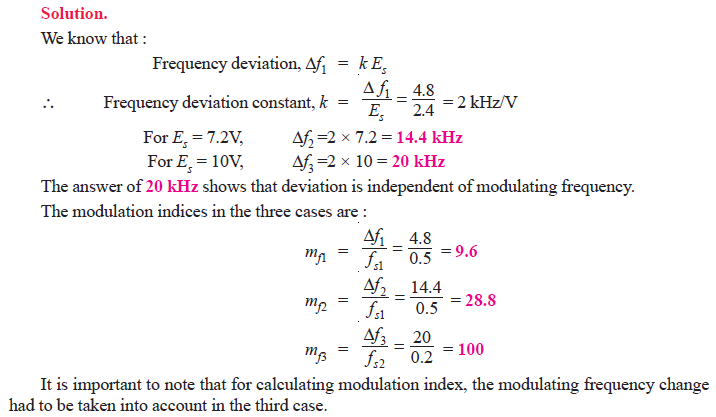Q1. The maximum peak-to-peak voltage of an AM wave is 16 mV and the minimum peak-to-peak voltage is 4 mV. Calculate the modulation factor.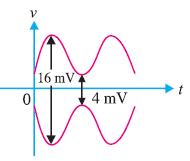Fig.1

Fig. 1 shows the conditions of the problem.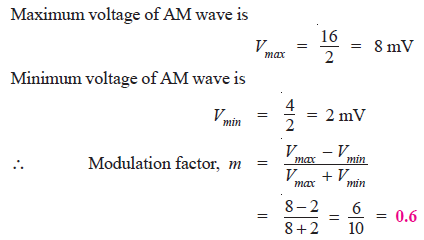Q2. A carrier of 100V and 1200 kHz is modulated by a 50 V, 1000 Hz sine wave signal. Find the modulation factor.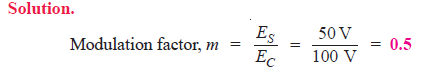Q3. An AM wave is represented by the expression :
v = 5 (1 + 0.6 cos 6280 t) sin 211 × 104 t volts
(i) What are the minimum and maximum amplitudes of the AM wave?
(ii) What frequency components are contained in the modulated wave and what is the amplitude
of each component?Q4. A sinusoidal carrier voltage of frequency 1 MHz and amplitude 100 volts is amplitude modulated by the sinusoidal voltage of frequency 5 kHz producing 50% modulation. Calculate the frequency and amplitude of lower and upper sideband terms.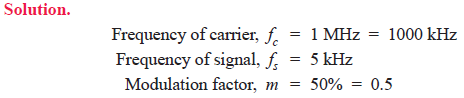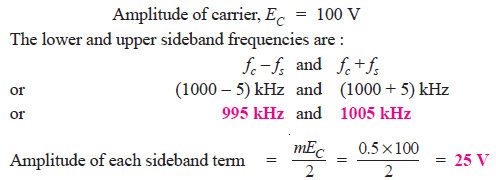Q5.A carrier wave of frequency 10 MHz and peak value 10V is amplitude modulated by a 5- kHz sine wave of amplitude 6V. Determine (i) modulation factor (ii) sideband frequencies and (iii) amplitude of sideband components. Draw the frequency spectrum.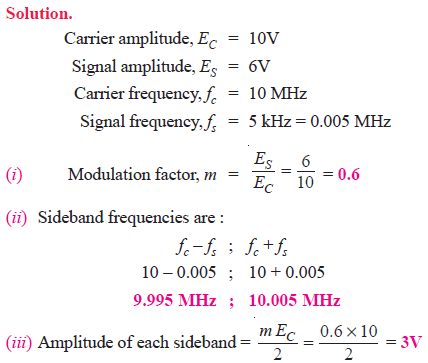Fig.2:

Fig. 2 shows the frequency spectrum of the A.M. wave.

Q6. A carrier wave of 500 watts is subjected to 100% amplitude modulation. Determine :
(i) power in sidebands (ii) power of the modulated wave.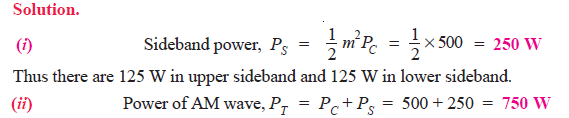Q7. A 50 kW carrier is to be modulated to a level of (i) 80% (ii) 10%. What is the total sideband power in each case ?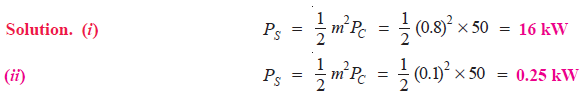Q8. A 40kW carrier is to be modulated to a level of 100%.
(i) What is the carrier power after modulation?
(ii) How much audio power is required if the efficiency of the modulated RF amplifier is 72%?

Solution. Fig. 3 shows the block diagram indicating the power relations.
(i) Since the carrier itself is unaffected by the modulating signal, there is no change in the carrier power level.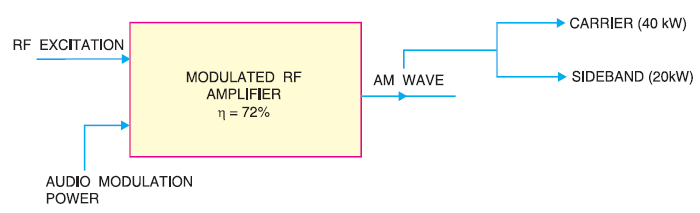Fig.3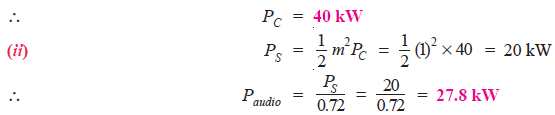Q9. An audio signal of 1 kHz is used to modulate a carrier of 500 kHz. Determine (i) sideband frequencies (ii) bandwidth required.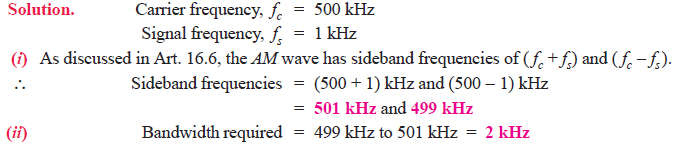Q10. The load current in the transmitting antenna of an unmodulated AM transmitter is 8A. What will be the antenna current when modulation is 40%?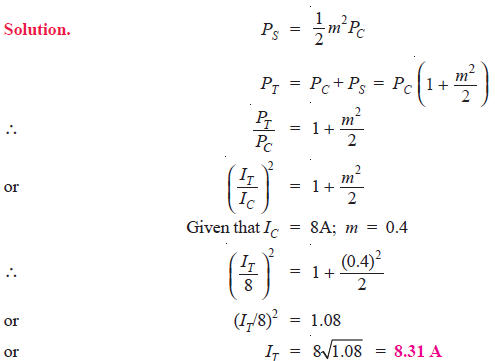Q11. The antenna current of an AM transmitter is 8A when only the carrier is sent but it increases to 8.93A when the carrier is sinusoidally modulated. Find the % age modulation.

Solution.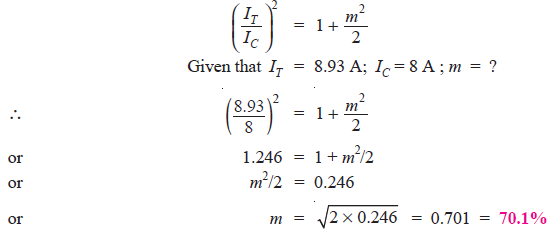Q12. The r.m.s. value of carrier voltage is 100 V. After amplitude modulation by a sinusoidal a.f. voltage, the r.m.s. value becomes 110 V. Calculate the modulation index.Q13. An AM wave consists of the following components :
Carrier component = 5 V peak value
Lower sideband component = 2.5 V peak value
Upper sideband component = 2.5 V peak value
If the AM wave drives a 2 kΩ resistor, find the power delivered to the resistor by (i) carrier (ii)
lower sideband component and (iii) upper sideband component. What is the total power delivered?

Solution. Fig. 4 (i) shows the frequency spectrum of AM wave whereas Fig. 4(ii) shows the equivalent circuit.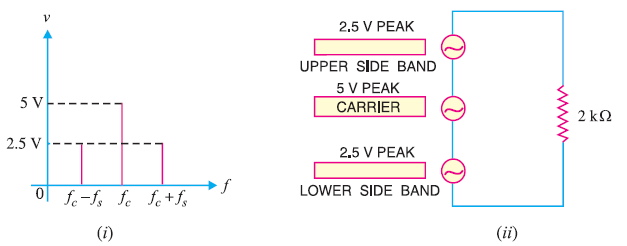Fig.4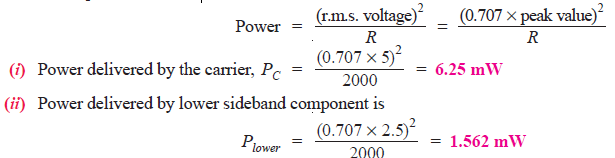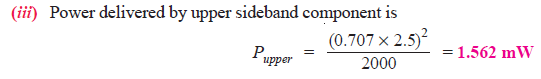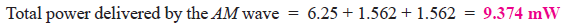Q14. A frequency-modulated voltage wave is given by the equation :
e = 12 cos (6 × 108t + 5 sin 1250 t)
Find (i) carrier frequency (ii) signal frequency (iii) modulation index (iv) maximum frequency deviation (v) power dissipated by the FM wave in a 10-ohm resistor.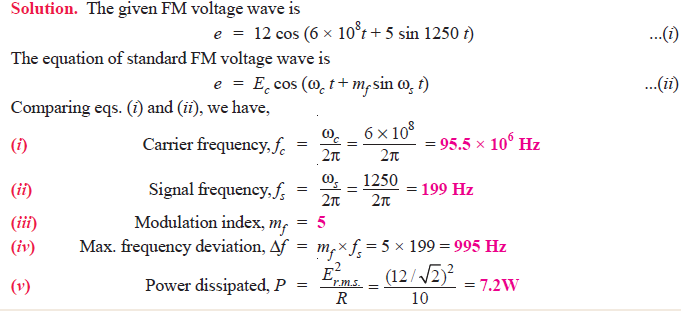Q15. A 25 MHz carrier is modulated by a 400 Hz audio sine wave. If the carrier voltage is 4V and the maximum frequency deviation is 10 kHz, write down the voltage equation of the FM wave.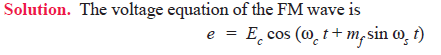Q16. Calculate the modulation index for an FM wave where the maximum frequency deviation is 50 kHz and the modulating frequency is 5 kHz.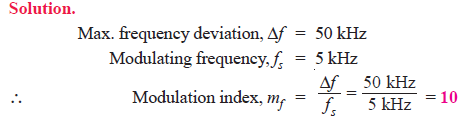Q17. The carrier frequency in an FM modulator is 1000 kHz. If the modulating frequency is 15 kHz, what are the first three upper sideband and lower sideband frequencies?Q18. In a frequency modulated wave, the frequency deviation constant is 75 kHz/volt and the signal amplitude is 2V. Find the maximum frequency deviation.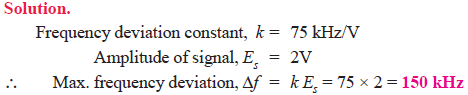Q19. In an FM system, when the audio frequency (AF) is 500 Hz and the AF voltage is 2.4V, the frequency deviation is 4.8 kHz. If the AF voltage is now increased to 7.2V, what is the new frequency deviation? If the AF voltage is raised to 10V while the AF is dropped to 200 Hz, what is the deviation? Find the modulation index in each case.